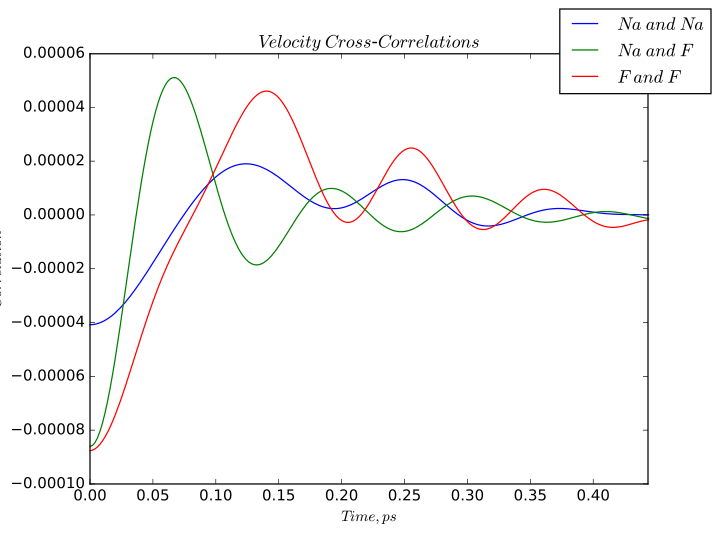$$\renewcommand\AA{\unicode{x212B}}$$

# VelocityCrossCorrelations v1¶

## Summary¶

Imports trajectory data from an nMoldyn-generated .nc file and calculates velocity cross-correlations between atomic species. The algorithm calculates the velocity cross-correlation of every pair of particles and averages the correlations according to the particles’ atomic species. Timestep must be specified in femtoseconds.

## Properties¶

Name

Direction

Type

Default

Description

InputFile

Input

string

Mandatory

Input .nc file with an MMTK trajectory

Timestep

Input

string

1.0

Specify the timestep between trajectory points in the simulation, fs

OutputWorkspace

Output

Workspace

Mandatory

Output workspace name

## Description¶

Loads a netcdf file generated by nMoldyn containing MMTK format trajectories. The algorithm calculates velocity cross-correlations of each pair of particles, sums and averages the correlations into bins according to the type of pairing. The correlations are also scaled by coherent scattering lengths of different atom types.

## Example¶

Velocity cross-correlations calculated for sodium fluoride.## Usage¶

CrossCorr = VelocityCrossCorrelations(InputFile = 'trajectories.nc',
Timestep = '2.0')


Categories: AlgorithmIndex | Simulation

## Source¶

Python: VelocityCrossCorrelations.py